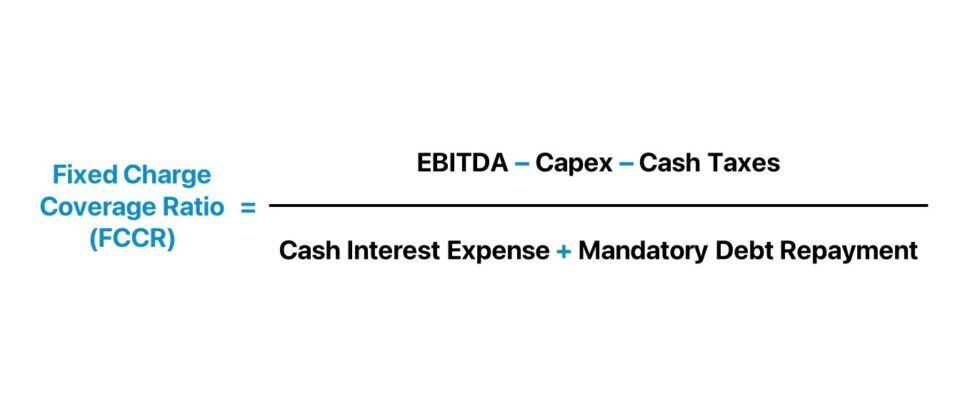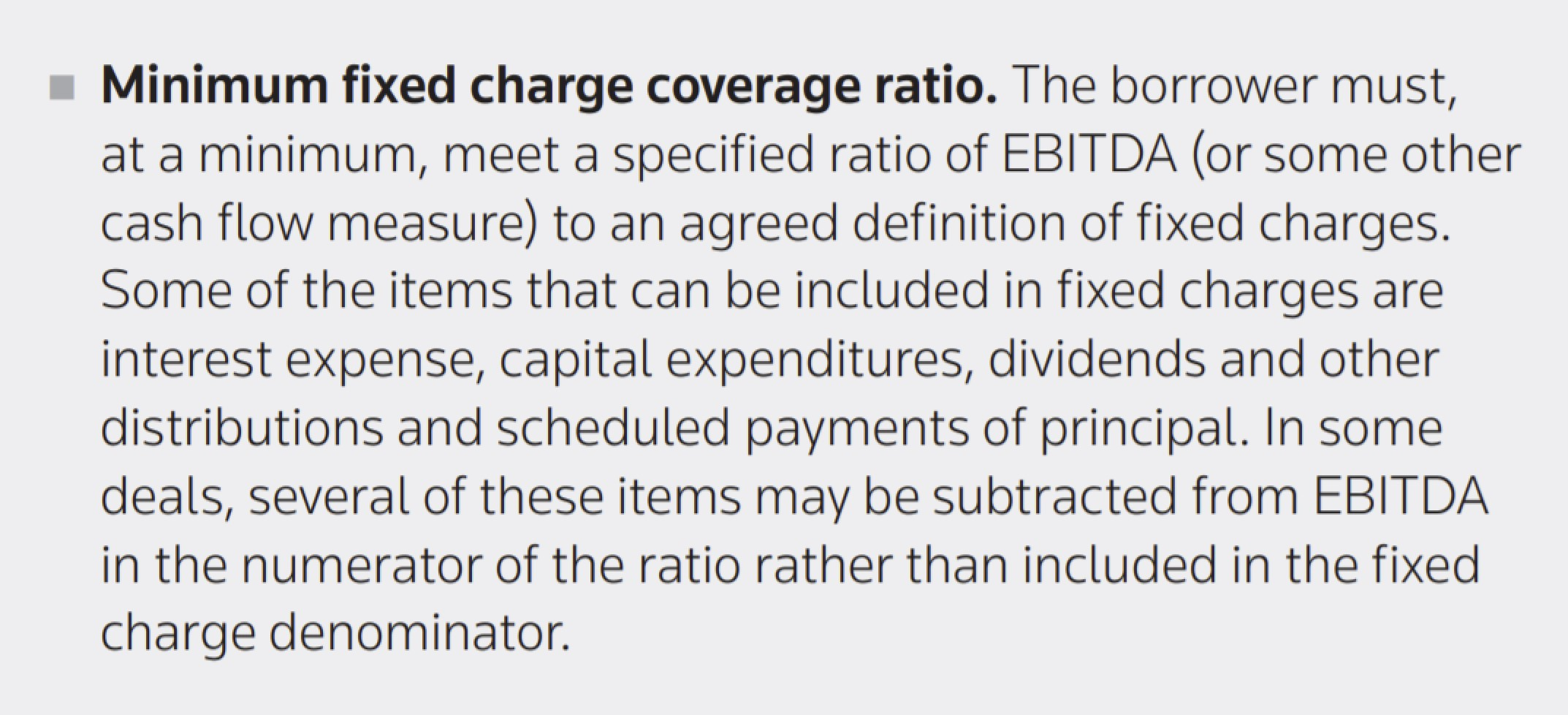Welcome to Wall Street Prep! Use code at checkout for 15% off.# Fixed Charge Coverage Ratio (FCCR)

Guide to Understanding the Fixed Charge Coverage Ratio (FCCR)## How to Calculate FCCR?

The fixed charge coverage ratio (FCCR) is a solvency ratio that assesses if a company’s cash flows are adequate to meet its fixed charges.

The fixed charge coverage ratio (FCCR) answers the question:

• “Does the company generate enough cash flow to meet its fixed charges?”

Conceptually, the FCCR represents the number of times a company could hypothetically pay off its annual fixed charges.

Oftentimes, lenders utilize the FCCR to determine the creditworthiness of a potential or existing borrower.

Classifying costs as fixed charges requires some discretion, but in general, they must meet the following criteria:

• Predictable: The cost should be recurring and quantified with minimal variance.
• Non-Discretionary: The cost should either directly (or indirectly) contribute toward the company’s revenue generation and business model, i.e. applicable to the day-to-day operations.
• Fixed Costs: The charge should not fluctuate based on the amount of revenue, unlike variable costs.

For example, the amount due and the dates when interest expense and mandatory debt repayment come due are outlined in the loan agreement – in addition, the debt associated with these cash outflows was issued to fund operations (or related functions), and the costs were pre-negotiated (i.e. fixed).

## FCCR Formula

Broadly, the fixed charge coverage ratio (FCCR) is a ratio that compares an earnings metric to the total fixed charges.

There are two common approaches to calculating the FCCR, which we’ll refer to as the “GAAP” and “Non-GAAP” variations for simplicity.

The first method abides closer to GAAP accounting and divides a company’s earnings before interest and taxes (EBIT) by fixed charges before taxes plus interest expense.

Fixed Charge Coverage Ratio = (EBIT + Fixed Charges Before Taxes) ÷ (Fixed Charges Before Taxes + Interest Expense)

Suppose that a company has the following financials.

• EBIT = \$250,000
• Fixed Charges = \$150,000
• Interest Payments = \$10,000

The numerator is equal to \$450,000 (\$250,000 + \$150,000), whereas the denominator is equal to \$160,000 (\$150,000 + \$10,000).

• FCCR = \$450,000 ÷ \$160,000 = 2.5x

However, EBIT is a GAAP measure of profitability – thus, many equity analysts adjust the metric given the drawbacks of accrual accounting, such as the inclusion of non-cash items, most notably depreciation and amortization (D&A).

That being said, the second approach for calculating FCCR starts with a non-GAAP metric, earnings before interest, taxes, depreciation, and amortization (EBITDA).

In the non-GAAP approach, FCCR is calculated as the ratio between.

Capex is subtracted while D&A is added back (i.e. EBITDA) since Capex is a real cash outflow, but D&A is a non-cash expense related to accrual accounting.

Fixed Charge Coverage Ratio = (EBITDACapexCash Taxes) ÷ (Cash Interest Expense + Mandatory Debt Amortization)

EBITDA is already a non-GAAP metric, yet in this context, it can be further changed by discretionary adjustments – as long as there is an agreement in writing allowing as such between the borrower and lender(s).

The latter non-GAAP EBITDA approach is far more common in practice, whereas the GAAP EBIT approach is more often taught in academia.

Besides interest and mandatory principal amortization, the following charges could also be included if deemed appropriate:

• Lease Payments
• Preferred Dividends
###### Discretionary and Non-Cash Spending

In the FCCR calculation, growth Capex or optional prepayment of debt should be excluded since they constitute discretionary spending.

PIK interest and deferred taxes should also be excluded because they are non-cash (i.e. no real cash outflow occurred).

## What is a Good FCCR?

Like the interest coverage ratio – i.e. the times interest earned (TIE) ratio – the higher the ratio, the better the company’s creditworthiness.

• FCCR = 2x → Can Pay Off Fixed Charges Twice
• FCCR = 1x → Can Pay Off Fixed Charges Once
• FCCR < 1x → Cannot Pay Off Fixed Charges

The higher FCCR, the stronger the company’s creditworthiness as a borrower – all else being equal.

Companies with higher FCCRs have less of their earnings spent on fixed charges like interest, leases, and principal repayments — therefore, more free cash flows (FCFs) remain.

Further, higher FCFs reduce the borrower’s risk of missing a scheduled payment to a third party and allow for more re-investments and discretionary spending to drive growth.

## What is the Minimum FCCR in Loan Covenants?

Certain lending agreements contain covenants based in part on the fixed charge coverage ratio (FCCR).

The so-called “FCCR minimum” frequently appears in secured credit facilities, e.g. ABL revolvers and senior term loans.

The FCCR covenant forces the borrower to maintain certain metrics above a specified threshold – remember, a lower FCCR puts a greater risk on the lenders.

The minimum fixed charge coverage ratio (FCCR) is typically set around 1.0x to 1.25x.

If the FCCR declines below 1.0x, the company will turn cash flow negative unless additional external financing is obtained – which in such a scenario, would likely be difficult.

However, lenders do not rely on the FCCR by itself, as the FCCR is just one of many credit metrics that help them understand the financial health of a company.FCCR Formal Definition (Source: Paul Weiss)

## What is the Difference Between FCCR vs. Times Interest Earned Ratio (TIE)?

Both FCCR and TIE conceptually have the same objective, i.e. deciding if the company has adequate earnings to meet certain payments.

Since more costs like the annual lease payment are accounted for, the FCCR is a relatively more conservative measure of a company’s ability to meet its upcoming payments.

In effect, the FCCR is inherently a more conservative calculation, which is why senior, risk-averse lenders are typically the ones that pay close attention to the metric.

## FCCR Calculator

We’ll now move to a modeling exercise, which you can access by filling out the form below.#### Excel TemplateSubmitting...

## FCCR Calculation Example

In our illustrative example, we’ll calculate a company’s fixed charge coverage ratio (FCCR) using the following assumptions.

• EBITDA = \$20 million
• Capex = \$2.5 million
• Cash Taxes = \$5 million
• Interest Expense = \$2.25 million
• Mandatory Debt Repayment = \$4 million

After subtracting Capex and cash taxes from EBITDA, we’re left with \$12.5 million for the covenant-adjusted EBITDA, i.e. the lender-negotiated earnings amount that the covenant is set upon.

• Covenant Adjusted EBITDA = \$20 million – \$2.5 million – \$5 million = \$12.5 million

In the next step, we will add our two fixed charges – the interest expense and mandatory debt repayment – for a total fixed charges amount of \$6.25 million.

• Total Fixed Charges = \$2.25 million + \$4 million = \$6.25 million

In the final step, we can now calculate the fixed charge coverage ratio by dividing the Covenant Adjusted EBITDA by the Total Fixed Charges.

• Fixed Charge Coverage Ratio = \$12.5 million ÷ \$6.25 million = 2.0x

In this case, the 2.0x FCCR suggests the Company’s earnings are sufficiently adequate to pay off its total fixed charges two times.Step-by-Step Online Course

### Everything You Need To Master Financial Modeling

Enroll in The Premium Package: Learn Financial Statement Modeling, DCF, M&A, LBO and Comps. The same training program used at top investment banks.Inline FeedbacksLearn Financial Modeling Online

Everything you need to master financial and valuation modeling: 3-Statement Modeling, DCF, Comps, M&A and LBO.

X

The Wall Street Prep Quicklesson Series

7 Free Financial Modeling Lessons

Get instant access to video lessons taught by experienced investment bankers. Learn financial statement modeling, DCF, M&A, LBO, Comps and Excel shortcuts.